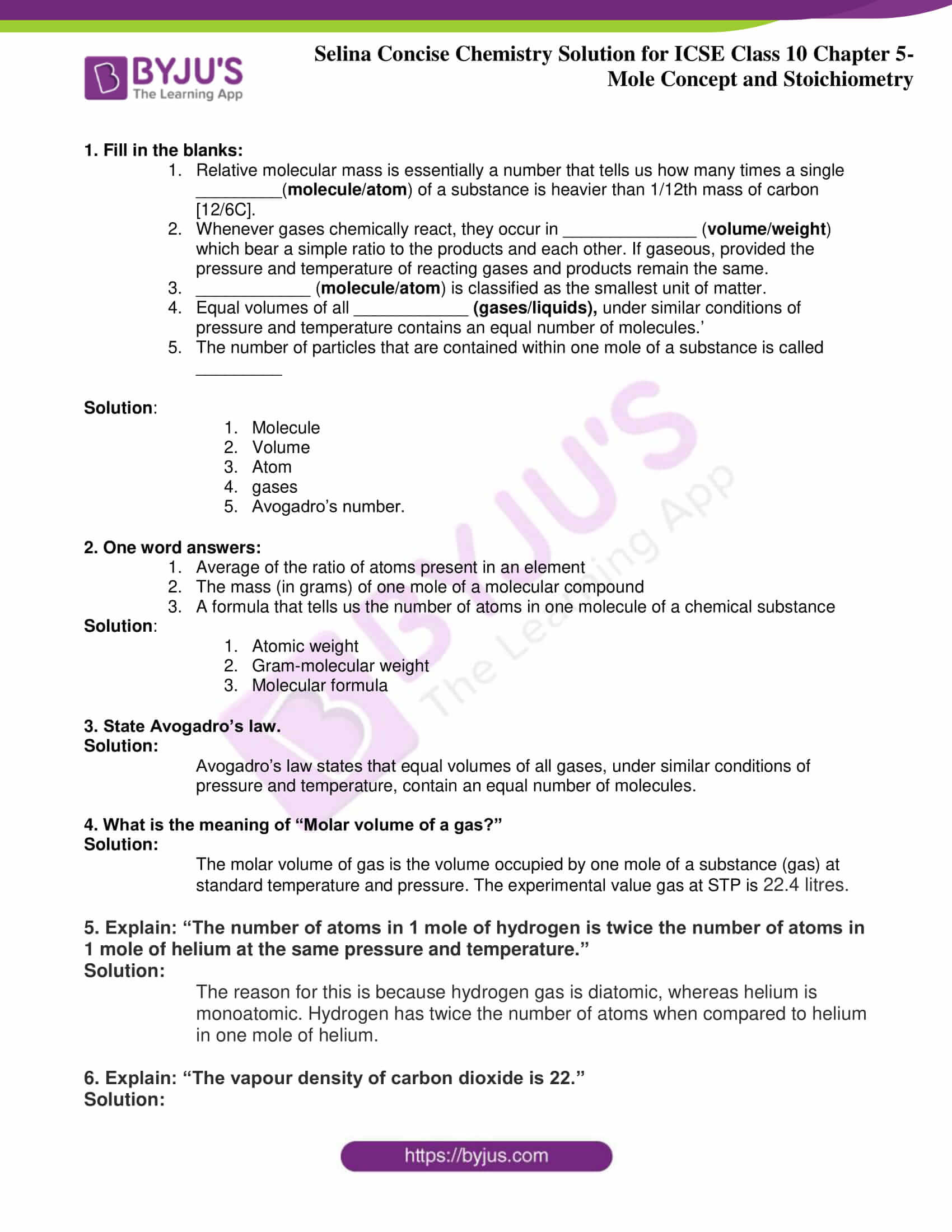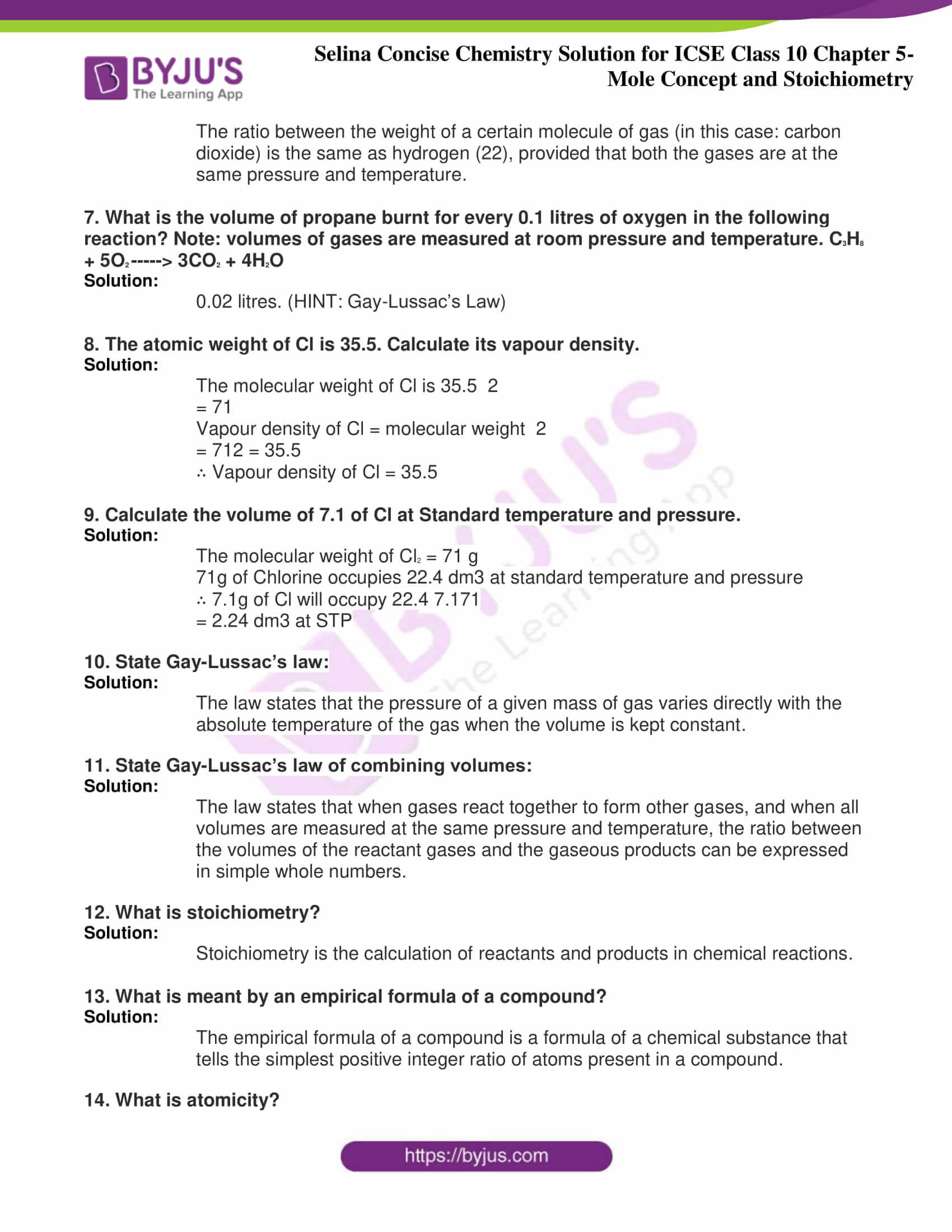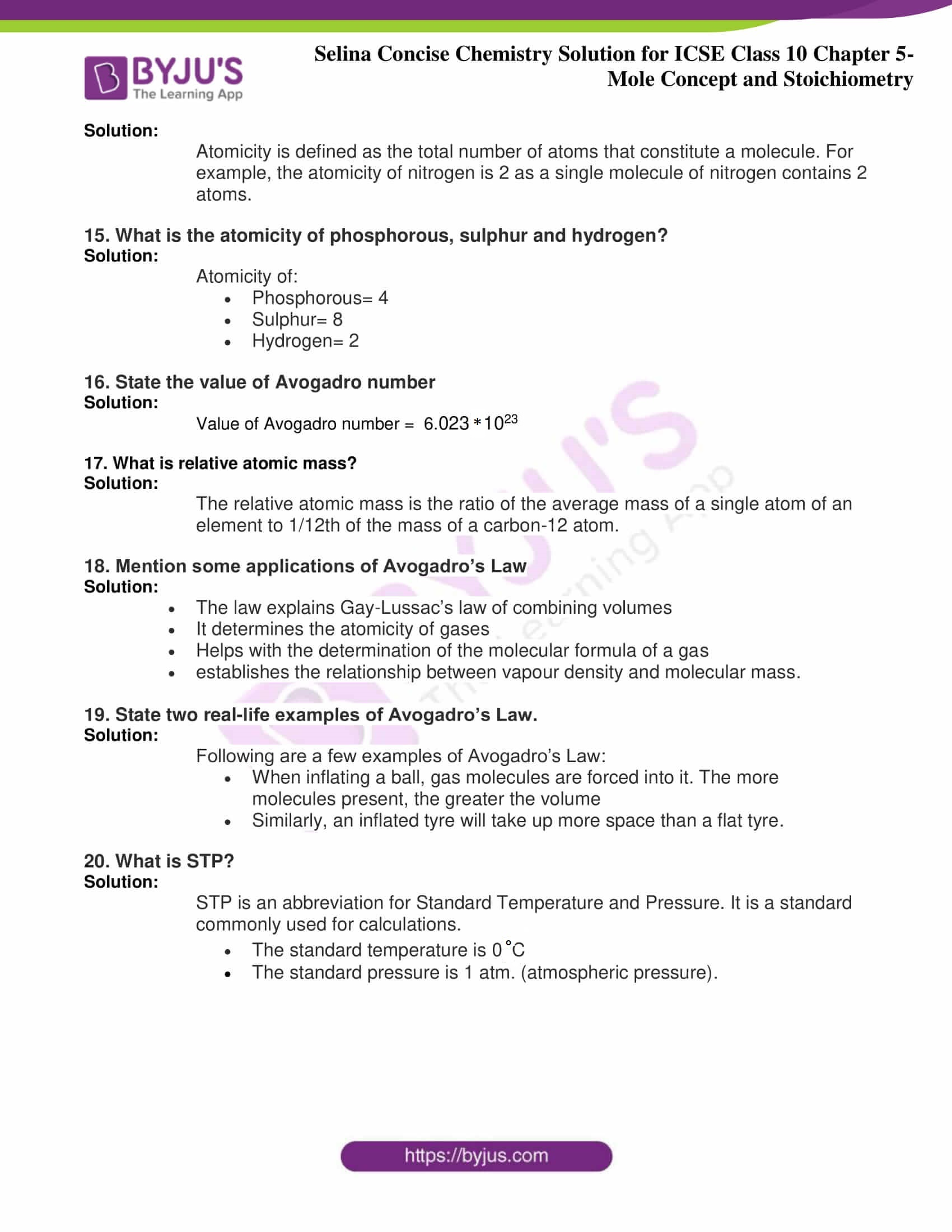# Selina Concise Chemistry Solution for ICSE Class 10 Chapter 5 Mole concept and Stoichiometry

One of the most fundamental ideas in class 10 Selina chemistry textbook is the mole concept. In chapter 5, students will basically acquire knowledge on how to use the mole concept in order to relate amounts of chemicals to each other or the process of stoichiometry. They will also understand things like the meaning of molar and atomic mass, empirical and molecular formulas, limiting reagents, and students will have to solve some problems related to the concepts. As students need to be thorough with the chapter in order to succeed in the exams, we are offering concise Chemistry class 10 ICSE solutions for chapter 5 here for free.

## Download PDF Of Selina Concise Chemistry Solution for ICSE Class 10 Chapter 5### Access Selina Concise Chemistry Solution for ICSE Class 10 Chapter 5

1. Fill in the blanks:

1. Relative molecular mass is essentially a number that tells us how many times a single _________(molecule/atom) of a substance is heavier than 1/12th mass of carbon [12/6C].
2. Whenever gases chemically react, they occur in ______________ (volume/weight) which bear a simple ratio to the products and each other. If gaseous, provided the pressure and temperature of reacting gases and products remain the same.
3. ____________ (molecule/atom) is classified as the smallest unit of matter.
4. Equal volumes of all ____________ (gases/liquids), under similar conditions of pressure and temperature contains an equal number of molecules.’
5. The number of particles that are contained within one mole of a substance is called _________

Solution:

1. Molecule
2. Volume
3. Atom
4. gases

1. Average of the ratio of atoms present in an element
2. The mass (in grams) of one mole of a molecular compound
3. A formula that tells us the number of atoms in one molecule of a chemical substance

Solution:

1. Atomic weight
2. Gram-molecular weight
3. Molecular formula

Solution:

Avogadro’s law states that equal volumes of all gases, under similar conditions of pressure and temperature, contain an equal number of molecules.

4. What is the meaning of “Molar volume of a gas?”

Solution:

The molar volume of gas is the volume occupied by one mole of a substance (gas) at standard temperature and pressure. The experimental value gas at STP is 22.4 litres.

5. Explain: “The number of atoms in 1 mole of hydrogen is twice the number of atoms in 1 mole of helium at the same pressure and temperature.”

Solution:

The reason for this is because hydrogen gas is diatomic, whereas helium is monoatomic. Hydrogen has twice the number of atoms when compared to helium in one mole of helium.

6. Explain: “The vapour density of carbon dioxide is 22.”

Solution:

The ratio between the weight of a certain molecule of gas (in this case: carbon dioxide) is the same as hydrogen (22), provided that both the gases are at the same pressure and temperature.

7. What is the volume of propane burnt for every 0.1 litres of oxygen in the following reaction? Note: volumes of gases are measured at room pressure and temperature. C3H8 + 5O2 —–> 3CO2 + 4H2O

Solution:

0.02 litres. (HINT: Gay-Lussac’s Law)

8. The atomic weight of Cl is 35.5. Calculate its vapour density.

Solution:

The molecular weight of Cl is 35.5 2

= 71

Vapour density of Cl = molecular weight 2

= 712 = 35.5

∴ Vapour density of Cl = 35.5

9. Calculate the volume of 7.1 of Cl at Standard temperature and pressure.

Solution:

The molecular weight of Cl2 = 71 g

71g of Chlorine occupies 22.4 dm3 at standard temperature and pressure

∴ 7.1g of Cl will occupy 22.4 7.171

= 2.24 dm3 at STP

10. State Gay-Lussac’s law:

Solution:

The law states that the pressure of a given mass of gas varies directly with the absolute temperature of the gas when the volume is kept constant.

11. State Gay-Lussac’s law of combining volumes:

Solution:

The law states that when gases react together to form other gases, and when all volumes are measured at the same pressure and temperature, the ratio between the volumes of the reactant gases and the gaseous products can be expressed in simple whole numbers.

12. What is stoichiometry?

Solution:

Stoichiometry is the calculation of reactants and products in chemical reactions.

13. What is meant by an empirical formula of a compound?

Solution:

The empirical formula of a compound is a formula of a chemical substance that tells the simplest positive integer ratio of atoms present in a compound.

14. What is atomicity?

Solution:

Atomicity is defined as the total number of atoms that constitute a molecule. For example, the atomicity of nitrogen is 2 as a single molecule of nitrogen contains 2 atoms.

15. What is the atomicity of phosphorous, sulphur and hydrogen?

Solution:

Atomicity of:

• Phosphorous= 4
• Sulphur= 8
• Hydrogen= 2

16. State the value of Avogadro number

Solution:

Value of Avogadro number =  6.023 × 1023

17. What is relative atomic mass?

Solution:

The relative atomic mass is the ratio of the average mass of a single atom of an element to 1/12th of the mass of a carbon-12 atom.

18. Mention some applications of Avogadro’s Law

Solution:

• The law explains Gay-Lussac’s law of combining volumes
• It determines the atomicity of gases
• Helps with the determination of the molecular formula of a gas
• establishes the relationship between vapour density and molecular mass.

19. State two real-life examples of Avogadro’s Law.

Solution:

Following are a few examples of Avogadro’s Law:

• When inflating a ball, gas molecules are forced into it. The more molecules present, the greater the volume
• Similarly, an inflated tyre will take up more space than a flat tyre.

20. What is STP?

Solution:

STP is an abbreviation for Standard Temperature and Pressure. It is a standard commonly used for calculations.

• The standard temperature is 0°C
• The standard pressure is 1 atm. (atmospheric pressure).

These solutions have been designed by experts who have further used a very simple language and style to help students understand all the concepts, theories, formulas, etc. clearly. Students can download these solutions in the form of a PDF and use it as an effective study tool. With these, students can prepare well and even achieve better results in the board examinations.

The given solutions are as per the 2019-20 Concise Selina textbook. The Selina Solutions for the academic year 2020-21 will be updated soon.